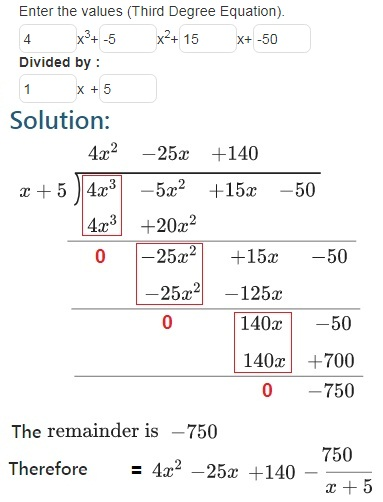# Polynomial Long Division Calculator

Enter the values (Third Degree Equation).

 Divided by : x3 + x2 + x + x +

Polynomial long division is a formula for dividing a polynomial by other polynomial of the identical or lower degree, the generalised form of the common arithmetic technique is called as long division.

It is used in manual methods of calculation , because it separates an otherwise complex division issue into smaller ones.

### Example